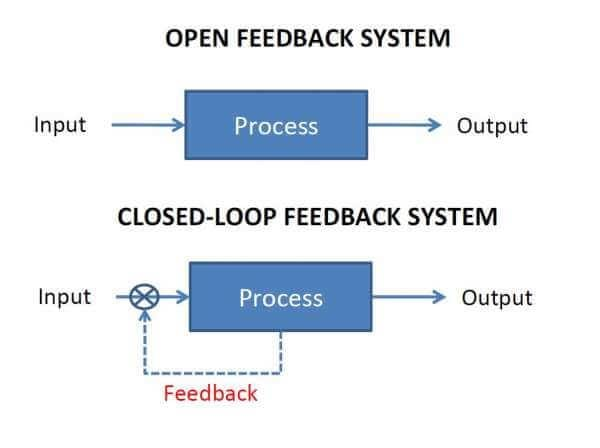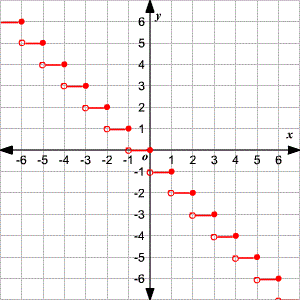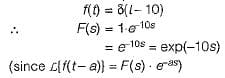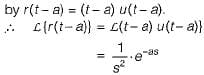# Test: Introduction to Control Systems

## 10 Questions MCQ Test Control Systems | Test: Introduction to Control Systems

Description
Attempt Test: Introduction to Control Systems | 10 questions in 30 minutes | Mock test for Electronics and Communication Engineering (ECE) preparation | Free important questions MCQ to study Control Systems for Electronics and Communication Engineering (ECE) Exam | Download free PDF with solutions
QUESTION: 1

### As compared to an open loop system, a closed loop system is:

Solution:

Open loop system always follows the input but closed loop system always reduces the error irrespective of the input applied. So closed loop system is more accurate but less stable.QUESTION: 2

### Linear system obeys:

Solution:

Linear system obeys both reciprocity theorem and principle of maximum power transfer.

QUESTION: 3

### The most commonly used input signal(s) in a control system is/are:

Solution:

A step function is commonly used as a input signal in a control system because:

• If we go through first order system, here we calculate steady-state error by providing input function then we got zero steady-state error.
• Actually in step function, output follows input always that means steady error is minimum.

Step Function:QUESTION: 4

The Laplace transform of a transportation Iag of 10 seconds is:

Solution:QUESTION: 5

Consider the following statements regarding control systems:
1. In open loop control system, the control action depends upon the desired output.
2. All control systems operated by present timing mechanism are open loop.
3. in a closed loop control system feedback signal is usually negative.
4. In open-loop control system stability cannot be ensured.

Q. Which of the above statements are correct?

Solution:
• There is no-control action in open loop systems. Hence, statement 1 is false.
• Generally, all control systems operated by present timing mechanism are open loop because they are more stable than closed loop control system. Hence, statement-2 is true.
• Due to various advantages of negative feedback systems (except reduction in gain), feedback signal is usually negative in a closed loop control system. Hence, statement-3 is true.
• In open-loop control systems stability can be ensured because they are more stable than closed-loop control system.
• Thus, only statements 2 and 3 are true.
QUESTION: 6

Assertion (A): Closed loop control systems are known as feedback control systems.
Reason (R): In closed loop control systems, the control action is dependent on the desired output.

Solution:
• In closed loop system there is the presence of a feedback path and this feedback path has connected from output of the system to the input of the system.
• The whole operation is controlled by the output of the system.
QUESTION: 7

As compared to a closed loop system, an open loop system is:

Solution:
• The main difference between an open-loop system and a closed-loop system is that the closed-loop system has the ability to self-correct while the open-loop system doesn't.
• Consequently, closed-loop systems are often called feedback control systems while open-loop systems are also known as non-feedback controls.
QUESTION: 8

Which of the following are the not characteristics of the closed loop systems?

Solution:

Feedback refers to the comparison of the final output to the desired output at respective input so as to get an accurate and error-free results and in the system improves the transient response of the system.

QUESTION: 9

Assertion (A): The control system which operates on the time basis is an open loop system.
Reason (R): A field control d.c. motor is an example of open loop system.

Solution:
• The control system which operates on the time basis is an open loop system. For example, in automatic control of traffic, the time for each lamp (red, yellow and green) is fixed.
• The operation of each lamp does not depends upon the density of the traffic but depends upon the fixed time. Hence, assertion is true.
• Reason is also true but, it is not the correct explanation of assertion.
QUESTION: 10

A ramp function occurs at t = a. It's Laplace transform is:

Solution:

Ramp function occurring at t = 0 is denoted by r(t) = tu(t).
Ramp function occurring at t - a will be denoted:Use Code STAYHOME200 and get INR 200 additional OFF Use Coupon Code